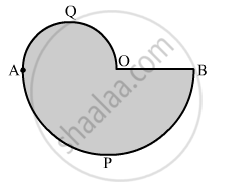Advertisement Remove all ads

# In the Given Figure, Apb and Aqo Are Semicircles and Ao = Ob. If the Perimeter of the Figure is 40 Cm, Find the Area of the Shaded Region. - Mathematics

Sum

In the given figure, APB and AQO are semicircles and AO = OB. If the perimeter of the figure is 40 cm, find the area of the shaded region.Advertisement Remove all ads

#### Solution

Permieter of shaded region = Length of the arc AQO + Length of the arc APB + Length of OB

=>40=1/2xx2pi("AO"/2) + 1/2xx2pi("OB") +"OB"

= 40 = 11/7"AO"+22/7"OB"+"OB"

=40 = 11/7"OB "+ 22/7 "OB" + "OB"         [∴ AO = OB]

=> 40 = 40/7 "OB"

⇒ OB =7 cm

Area of the shaded portion = Area of semicircle AQO + Area of semicircle APB

=1/2 pi(7/2)^2 + 1/2(7)^2

= 1/2 xx 22/7xx(7/2)^2+1/2xx22/7xx(7)^2

= 96.25 cm

Hence, the area of the shaded portion is 96.25 cm2.

Is there an error in this question or solution?
Advertisement Remove all ads

#### APPEARS IN

RS Aggarwal Secondary School Class 10 Maths
Chapter 18 Area of Circle, Sector and Segment
Exercise 18A | Q 40 | Page 823
Advertisement Remove all ads

#### Video TutorialsVIEW ALL 

Advertisement Remove all ads
Share
Notifications

View all notifications

Forgot password?